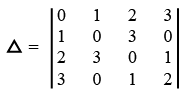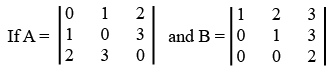# ISRO Scientist or Engineer Civil 2015

Instructions

For the following questions answer them individually

Question 71

# The value ofisQuestion 72

# The series $$1+\frac{1}{2^{2}}-\frac{1}{3^{2}}-\frac{1}{4^{2}}-\frac{1}{5^{2}}+\frac{1}{6^{2}}-\frac{1}{7^{2}}-\frac{1}{8^{2}}+............\infty$$ isQuestion 73

# The complete solution of the linear differential equation $$\frac{d^{2} y}{dx^{2}}+(a+b)\frac{dy}{dx}+aby=0$$Question 74

# To multiply a matrix by scalar K, multiplyQuestion 75

#Then the determinant AB has the valueQuestion 76

# The period of a simple pendulum is $$T = 2\pi \sqrt{\frac{L}{g}}$$.The maximum error in 7 due to the possible error upto 1% in ‘L’ and 2.5% in ‘g’ isQuestion 77

# The value of $$\int_{0}^{\frac{\pi}{2}} \sin^{2}\theta . \cos^{4}\theta d\theta$$ isQuestion 78

# If $$f(x, y) = x^{3}y - xy^{3}$$, then what is the value of $$[\frac{1}{(\frac{df}{dx})}+\frac{1}{(\frac{df}{dy})}] x = 1, y = 2 ?$$Question 79

# What is the complete solution for the equation $$x (y - z) p + y(z - x)q = z(x - y)?$$Question 80

# The solution of $$\frac{d^{2}z}{dx^{2}}+z = 0$$ given that when $$x = 0, z = e^{y}$$ and $$\frac{dz}{dx} = 1$$ isOR